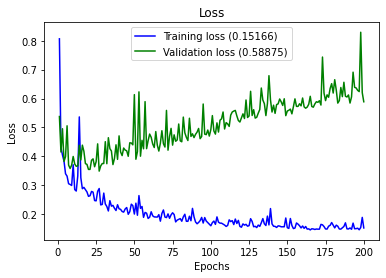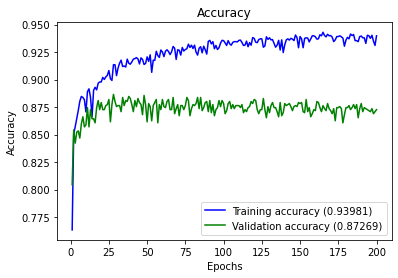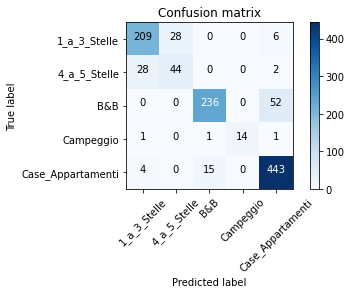# Multiclass Classification of Hotels# Multiclass Classification

by Ruslan Magana Vsevolodovna

Genova November 2020In this project we are going to classify the accommodations given by some features collected in one dataset. The target is the classification of the following types of accommodations:

1. Hotel 1-3 stars
2. Hotel 4+ stars
3. Bed & Breakfast
4. Campsites
5. Houses / apartments

### 1. Libraries

import pandas as pd

import numpy as np
import matplotlib.pyplot as plt
import itertools
from sklearn import datasets
from sklearn.preprocessing import LabelEncoder
from sklearn.model_selection import train_test_split, KFold, GridSearchCV
from sklearn.metrics import confusion_matrix, classification_report, accuracy_score
from keras.models import Sequential
from keras.layers import Dense
from keras.utils import to_categorical
from keras.wrappers.scikit_learn import KerasClassifier


### 2. Data Wrangling

df=pd.read_csv ('classification_dataset.csv',sep = '\t', )


Replace NaN Values with Zeros in Pandas DataFrame For an entire DataFrame using Pandas:

df=df.fillna(0)

df.head()

ID PROVINCIA COMUNE LOCALITA CAMERE SUITE LETTI BAGNI PRIMA_COLAZIONE IN_ABITATO ... ZONA_PERIFERICA ZONA_STAZIONE_FS ATTREZZATURE_VARIE CARTE_ACCETTATE LINGUE_PARLATE SPORT CONGRESSI LATITUDINE LONGITUDINE OUTPUT
0 0 BS PERTICA BASSA 0 2 0 4 1 0 0.0 ... 0.0 0.0 0 0 0 0 0 10.373501 45.751381 B&B
1 1 BS DESENZANO DEL GARDA 0 4 0 8 4 0 0.0 ... 0.0 0.0 0 0 0 0 0 10.538947 45.469485 B&B
2 2 BG BERGAMO 0 1 0 2 1 0 0.0 ... 0.0 0.0 Accettazione animali domestici 0 0 0 0 9.665355 45.704158 Case_Appartamenti
3 3 MN MANTOVA BOCCABUSA 93 2 194 96 0 0.0 ... 0.0 0.0 Ascensore,Ristorante,Bar,Accettazione animali ... American express,Visa,Master Card,Diners,Maest... Inglese,Francese,Spagnolo,Tedesco,Rumeno,Serbo... 0 Numero sale congressi 3,Congressi capacita' Mi... 10.828175 45.165506 4_a_5_Stelle
4 4 MI MILANO 0 2 0 4 1 0 0.0 ... 0.0 0.0 0 0 0 0 0 9.151637 45.457177 Case_Appartamenti

5 rows × 25 columns

There are some features that do not gives important information: Such as: ID, LOCALITA, LATITUDINE LONGITUDINE

del df['ID']
del df['LOCALITA']
del df['LATITUDINE']
del df['LONGITUDINE']
del df['PROVINCIA']
del df['COMUNE']

dfa=df


One-hot Encoding is a type of vector representation in which all of the elements in a vector are 0, except for one, which has 1 as its value, where 1 represents a boolean specifying a category of the element.

new_df = pd.concat([dfa.drop('ATTREZZATURE_VARIE', 1), dfa['ATTREZZATURE_VARIE'].str.get_dummies(sep=",")], 1)
new_df2 = pd.concat([new_df.drop('CARTE_ACCETTATE', 1), new_df['CARTE_ACCETTATE'].str.get_dummies(sep=",")], 1)
new_df3 = pd.concat([new_df2.drop('LINGUE_PARLATE', 1), new_df2['LINGUE_PARLATE'].str.get_dummies(sep=",")], 1)
new_df4 = pd.concat([new_df3.drop('SPORT', 1), new_df3['SPORT'].str.get_dummies(sep=",")], 1)
new_df5 = pd.concat([new_df4.drop('CONGRESSI', 1), new_df4['CONGRESSI'].str.get_dummies(sep=",")], 1)


new_df5.describe()

CAMERE SUITE LETTI BAGNI PRIMA_COLAZIONE IN_ABITATO SUL_LAGO VICINO_ELIPORTO VICINO_AEREOPORTO ZONA_CENTRALE ... Numero sale congressi 17 Numero sale congressi 2 Numero sale congressi 21 Numero sale congressi 3 Numero sale congressi 4 Numero sale congressi 5 Numero sale congressi 6 Numero sale congressi 7 Numero sale congressi 8 Numero sale congressi 9
count 6775.000000 6775.000000 6775.000000 6775.000000 6775.000000 6775.000000 6775.000000 6775.000000 6775.000000 6775.000000 ... 6775.000000 6775.000000 6775.000000 6775.000000 6775.00000 6775.000000 6775.000000 6775.000000 6775.000000 6775.000000
mean 16.261550 0.455646 35.002509 13.620517 0.101402 0.083542 0.052546 0.000590 0.027897 0.099041 ... 0.000295 0.008413 0.000148 0.004428 0.00428 0.001624 0.001328 0.001181 0.000886 0.000738
std 35.859435 3.592147 93.538492 29.174499 0.301883 0.276721 0.223142 0.024293 0.164689 0.298739 ... 0.017180 0.091344 0.012149 0.066401 0.06529 0.040264 0.036426 0.034345 0.029748 0.027158
min 0.000000 0.000000 0.000000 0.000000 0.000000 0.000000 0.000000 0.000000 0.000000 0.000000 ... 0.000000 0.000000 0.000000 0.000000 0.00000 0.000000 0.000000 0.000000 0.000000 0.000000
25% 2.000000 0.000000 4.000000 1.000000 0.000000 0.000000 0.000000 0.000000 0.000000 0.000000 ... 0.000000 0.000000 0.000000 0.000000 0.00000 0.000000 0.000000 0.000000 0.000000 0.000000
50% 4.000000 0.000000 8.000000 3.000000 0.000000 0.000000 0.000000 0.000000 0.000000 0.000000 ... 0.000000 0.000000 0.000000 0.000000 0.00000 0.000000 0.000000 0.000000 0.000000 0.000000
75% 14.000000 0.000000 25.000000 12.000000 0.000000 0.000000 0.000000 0.000000 0.000000 0.000000 ... 0.000000 0.000000 0.000000 0.000000 0.00000 0.000000 0.000000 0.000000 0.000000 0.000000
max 528.000000 110.000000 1816.000000 448.000000 1.000000 1.000000 1.000000 1.000000 1.000000 1.000000 ... 1.000000 1.000000 1.000000 1.000000 1.00000 1.000000 1.000000 1.000000 1.000000 1.000000

8 rows × 308 columns

Encode The Output Variable The output variable contains different string values.

When modeling multi-class classification problems using neural networks, it is good practice to reshape the output attribute from a vector that contains values for each class value to be a matrix with a boolean for each class value and whether or not a given instance has that class value or not.

new_df6 = pd.concat([new_df5.drop('OUTPUT', 1), new_df5['OUTPUT'].str.get_dummies(sep=",")], 1)

new_df6.dtypes

CAMERE               int64
SUITE                int64
LETTI                int64
BAGNI                int64
PRIMA_COLAZIONE      int64
...
1_a_3_Stelle         int64
4_a_5_Stelle         int64
B&B                  int64
Campeggio            int64
Case_Appartamenti    int64
Length: 313, dtype: object


We have now all our fetures numeric we can use it.

We have several fetures. we can REDUCE the dimensions by using Principal Component Analysis ( PCA )but for lack of time, we just show the standard procedure to classify with the current status of the dataset.

## 3. MODEL CREATION

dataset=new_df6.to_numpy()

X = dataset[:,0:308].astype(float)

len(X)

6775

Y = dataset[:,308:]

len(Y)

6775


The Keras library provides wrapper classes to allow you to use neural network models developed with Keras in scikit-learn.

There is a KerasClassifier class in Keras that can be used as an Estimator in scikit-learn, the base type of model in the library. The KerasClassifier takes the name of a function as an argument. This function must return the constructed neural network model, ready for training.

Below is a function that will create a baseline neural network for the Accommodation classification problem. It creates a simple fully connected network with one hidden layer that contains 616 neurons.

The hidden layer uses a rectifier activation function which is a good practice. Because we used a one-hot encoding for our dataset, the output layer must create 5 output values, one for each class. The output value with the largest value will be taken as the class predicted by the model.

The network topology of this simple one-layer neural network can be summarized as:

308 inputs -> [616 hidden nodes] -> 5 outputs

Note that we use a “softmax” activation function in the output layer. This is to ensure the output values are in the range of 0 and 1 and may be used as predicted probabilities.

Finally, the network uses the efficient Adam gradient descent optimization algorithm with a logarithmic loss function, which is called “categorical_crossentropy” in Keras.

seed = 7

x_train, x_test, y_train, y_test = train_test_split(X, Y, train_size=0.8, random_state=seed)

x_train, x_val, y_train, y_val = train_test_split(x_train, y_train, train_size=0.8, random_state=seed)


The hyperparameteres were computed in the optional section by using GridCSV

Best: 0.880295 using {‘batch_size’: 80, ‘epochs’: 200}

model = Sequential()
loss='categorical_crossentropy',
metrics=['accuracy'])


history = model.fit(x_train,
y_train,
epochs = 200,
batch_size = 80,
verbose=0,
validation_data=(x_val,y_val))


## 4. RESULTS

We define some programs to visualize the results Plot History : plot loss and accuracy from the history Full Report : print a full report and plot a confusion matrix

def plot_history(history):
loss_list = [s for s in history.history.keys() if 'loss' in s and 'val' not in s]
val_loss_list = [s for s in history.history.keys() if 'loss' in s and 'val' in s]
acc_list = [s for s in history.history.keys() if 'acc' in s and 'val' not in s]
val_acc_list = [s for s in history.history.keys() if 'acc' in s and 'val' in s]

if len(loss_list) == 0:
print('Loss is missing in history')
return

## As loss always exists
epochs = range(1,len(history.history[loss_list]) + 1)

## Loss
plt.figure(1)
for l in loss_list:
plt.plot(epochs, history.history[l], 'b', label='Training loss (' + str(str(format(history.history[l][-1],'.5f'))+')'))
for l in val_loss_list:
plt.plot(epochs, history.history[l], 'g', label='Validation loss (' + str(str(format(history.history[l][-1],'.5f'))+')'))

plt.title('Loss')
plt.xlabel('Epochs')
plt.ylabel('Loss')
plt.legend()

## Accuracy
plt.figure(2)
for l in acc_list:
plt.plot(epochs, history.history[l], 'b', label='Training accuracy (' + str(format(history.history[l][-1],'.5f'))+')')
for l in val_acc_list:
plt.plot(epochs, history.history[l], 'g', label='Validation accuracy (' + str(format(history.history[l][-1],'.5f'))+')')

plt.title('Accuracy')
plt.xlabel('Epochs')
plt.ylabel('Accuracy')
plt.legend()
plt.show()

def plot_confusion_matrix(cm, classes,
normalize=False,
cmap=plt.cm.Blues):
"""
This function prints and plots the confusion matrix.
Normalization can be applied by setting normalize=True.
"""
if normalize:
cm = cm.astype('float') / cm.sum(axis=1)[:, np.newaxis]
title='Normalized confusion matrix'
else:
title='Confusion matrix'

plt.imshow(cm, interpolation='nearest', cmap=cmap)
plt.title(title)
plt.colorbar()
tick_marks = np.arange(len(classes))
plt.xticks(tick_marks, classes, rotation=45)
plt.yticks(tick_marks, classes)

fmt = '.2f' if normalize else 'd'
thresh = cm.max() / 2.
for i, j in itertools.product(range(cm.shape), range(cm.shape)):
plt.text(j, i, format(cm[i, j], fmt),
horizontalalignment="center",
color="white" if cm[i, j] > thresh else "black")

plt.tight_layout()
plt.ylabel('True label')
plt.xlabel('Predicted label')
plt.show()

## multiclass or binary report
## If binary (sigmoid output), set binary parameter to True
def full_multiclass_report(model,
x,
y_true,
classes,
batch_size=32,
binary=False):

# 1. Transform one-hot encoded y_true into their class number
if not binary:
y_true = np.argmax(y_true,axis=1)

# 2. Predict classes and stores in y_pred
y_pred = model.predict_classes(x, batch_size=batch_size)

# 3. Print accuracy score
print("Accuracy : "+ str(accuracy_score(y_true,y_pred)))

print("")

# 4. Print classification report
print("Classification Report")
print(classification_report(y_true,y_pred,digits=5))

# 5. Plot confusion matrix
cnf_matrix = confusion_matrix(y_true,y_pred)
print(cnf_matrix)
plot_confusion_matrix(cnf_matrix,classes=classes)


### Results

plot_history(history)labels=list(new_df6.columns)[308:]

labels

['1_a_3_Stelle', '4_a_5_Stelle', 'B&B', 'Campeggio', 'Case_Appartamenti']

full_multiclass_report(model,
x_val,
y_val,
labels
)

WARNING:tensorflow:From <ipython-input-30-3b4d949a528c>:47: Sequential.predict_classes (from tensorflow.python.keras.engine.sequential) is deprecated and will be removed after 2021-01-01.
Instructions for updating:
Please use instead:* np.argmax(model.predict(x), axis=-1),   if your model does multi-class classification   (e.g. if it uses a softmax last-layer activation).* (model.predict(x) > 0.5).astype("int32"),   if your model does binary classification   (e.g. if it uses a sigmoid last-layer activation).
Accuracy : 0.8726937269372693

Classification Report
precision    recall  f1-score   support

0    0.86364   0.86008   0.86186       243
1    0.61111   0.59459   0.60274        74
2    0.93651   0.81944   0.87407       288
3    1.00000   0.82353   0.90323        17
4    0.87897   0.95887   0.91718       462

accuracy                        0.87269      1084
macro avg    0.85804   0.81131   0.83182      1084
weighted avg    0.87443   0.87269   0.87164      1084

[[209  28   0   0   6]
[ 28  44   0   0   2]
[  0   0 236   0  52]
[  1   0   1  14   1]
[  4   0  15   0 443]]The results can be improved by using PCA and a Grid Search. GridSearchCV resolve the issue relative to the multiclass models when using custom scoring, find the best hyperparameters.

from keras.models import Sequential
from keras.layers import Dense
from keras.utils import np_utils
from keras.wrappers.scikit_learn import KerasClassifier
import numpy
import pandas as pd
from sklearn.preprocessing import LabelEncoder
from sklearn.model_selection import GridSearchCV

def create_model():
# create model
model = Sequential()
# Compile model
return model


# fix random seed for reproducibility
seed = 7
numpy.random.seed(seed)

# create model
model = KerasClassifier(build_fn=create_model, verbose=0)
# define the grid search parameters
batch_size = [5, 10, 20, 40, 60, 80, 100]
epochs = [10, 50, 100, 200]
param_grid = dict(batch_size=batch_size, epochs=epochs)
grid = GridSearchCV(estimator=model, param_grid=param_grid, n_jobs=1)

from sklearn.utils import shuffle

X, Y = shuffle(X, Y)

grid_result = grid.fit(X, Y)

# summarize results
print("Best: %f using %s" % (grid_result.best_score_, grid_result.best_params_))
means = grid_result.cv_results_['mean_test_score']
stds = grid_result.cv_results_['std_test_score']
params = grid_result.cv_results_['params']
for mean, stdev, param in zip(means, stds, params):
print("%f (%f) with: %r" % (mean, stdev, param))


Best: 0.880295 using {‘batch_size’: 80, ‘epochs’: 200}

which are the hyperparameters used before.

Congratulations! we classified with Neural Network the different types of accommodations.

Categories:

Posted: